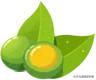# 坏字组词语 汉字坏怎么组词（清）（清水）

（情）（心情）

（晴）（晴天）

（请）（请客）

（睛）（眼睛）

（精）（精神）

（灯）（电灯）

（顶）（顶部）

（打）（打听）

（订）（订正）

（跑）（跑步）

（泡）（泡泡）

（抱）（拥抱）

（苞）（花苞）

（饱）（饱满）

（浇）（浇水）

（烧）（发烧）

（饶）（富饶）

（挠）（阻挠）

（晓）（知晓）

（翘）（翘尾巴）

（仔）（仔细）

（字）（写字）

（好）（好坏）

（披）（雨披）

（坡）（山坡）

（波）（水波）

（破）（破坏）

（疲）（疲劳）

（海）（大海）

（梅）（梅花）

（悔）（后悔）

（莓）（草莓）

（跟）（脚跟）

（根）（树根）

（退）（后退）

（眼）（眼睛）

（狠）（凶狠）

（很）（很好）

（恨）（仇恨）

（领）（带领）

（铃）（铃声）

（冷）（冰冷）

（岭）（山岭）

（龄）（年龄）

（河）（河水）

（何）（如何）

（奇）（奇怪）

（操）（操心）

（澡）（洗澡）

（燥）（干燥）

（噪）（噪音）

（油）（油亮）

（抽）（抽丝）

（邮）（邮局）

（庙）（寺庙）

（苗）（禾苗）

（细）（细心）

（思）（思想）

（雷）（打雷）

（累）（劳累）

（听）（听话）

（近）（近处）

（匠）（花匠）

（折）（折断）

（析）（分析）

（芹）（芹菜）

（跳）（跳高）

（桃）（桃花）

（挑）（挑选）

（逃）（逃走）

（眺）（眺望）

（漂）（漂亮）

（飘）（飘扬）

（瓢）（瓢虫）

（沙）（黄沙）

（纱）（纱布）

（吵）（吵闹）

（抄）（抄写）

（妙）（美妙）

（秒）（秒钟）

（拉）（拉手）

（粒）（颗粒）

（位）（座位）

（泣）（哭泣）

（妙）（美妙）

（秒）（秒钟）

（现）（现在）

（舰）（军舰）

（视）（电视）

（观）（观看）

（孩）（孩子）

（刻）（立刻）

（该）（应该）

（咳）（咳嗽）

（进）（进步）

（讲）（讲话）

（浪）（海浪）

（狼）（狼狗）

（娘）（姑娘）

（朗）（朗读）

（郎）（新郎）

（诗）（古诗）

（等）（等候）

（待）（等待）

（侍）（服侍）

（持）（坚持）

（机）（机会）

（饥）（饥饿）

（肌）（肌肉）

（讥）（讥笑）

（叽）（叽叽喳喳）

（什）（什么）

（计）（计划）

（叶）（树叶）

（汁）（果汁）

（伯）（伯父）

（拍）（拍手）

（怕）（害怕）

（红）（红色）

（虹）（彩虹）

（扛）（扛枪）

（池）（水池）

（地）（大地）

（他）（他们）

（她）（她们）

（秋）（秋天）

（伙）（伙伴）

（灭）（消灭）

（课）（上课）

（棵）（一棵树）

（颗）（颗粒）

（玩）（玩耍）

（园）（公园）

（完）（完成）

（远）（远近）

（点）（点头）

（战）（战斗）

（站）（站立）

（店）（书店）

（猫）（花猫）

（瞄）（瞄准）

（描）（描写）

（喵）（喵喵叫）

（球）（足球）

（救）（救命）

（把）（把手）

（爸）（爸爸）

（吧）（网吧）

（肥）（施肥）

（纪）（年纪）

（记）（忘记）

（起）（起床）

（妈）（妈妈）

（蚂）（蚂蚁）

（吗）（好吗）

（码）（码头）

（闯）（闯关）

（吹）（吹风）

（次）（次数）

（软）（柔软）

（欢）（欢乐）

（姐）（姐姐）

（祖）（祖国）

（组）（小组）

（阻）（阻止）

（活）（生活）

（话）（说话）

（刮）（刮风）

（括）（包括）

（适）（合适）

（蚜）（蚜虫）

（芽）（发芽）

（穿）（穿衣）

（鸦）（乌鸦）

（粉）（粉红）

（份）（月份）

（盼）（盼望）

（盆）（花盆）

（芬）（芬芳）

（纷）（大雪纷飞）

（愉）（愉快）

（偷）（小偷）

（喻）（比喻）

（榆）（榆树）

（放）（放学）

（房）（房间）

（访）（访问）

（防）（防守）

（仿）（模仿）

（芳）（芳香）

（纺）（纺织）

（样）（样子）

（痒）（挠痒痒）

（洋）（海洋）

（鲜）（海鲜）

（推）（推车）

（堆）（土堆）

（谁）（是谁）

（准）（准备）

（船）（船长）

（沿）（沿海）

（铅）（铅笔）

（到）（来到）

（室）（教室）

（屋）（房屋）

（挣）（挣钱）

（睁）（眼睁睁）

（筝）（风筝）

（净）（干净）

（证）（证明）

（征）（征服）

（政）（政府）

（喝）（喝水）

（渴）（口渴）

（揭）（揭发）

（葛）（葛根）

（错）（错误）

（惜）（爱惜）

（借）（借书）

（惊）（吃惊）

（景）（景色）

（凉）（凉快）

（忆）（回忆）

（艺）（艺术）

（亿）（亿万）

（级）（班级）

（极）（南极）

（圾）（垃圾）

（校）（学校）

（咬）（咬牙）

（郊）（郊外）

（较）（比较）

（胶）（胶水）

（桥）（木桥）

（骄）（骄傲）

（娇）（娇气）

（轿）（轿车）

（线）（直线）

（钱）（金钱）

（浅）（浅红）

（吓）（吓坏）

（虾）（河虾）

（杯）（杯子）

（坏）（好坏）

（怀）（关怀）

（理）（理发）

（狸）（狐狸）

（鲤）（鲤鱼）

（厘）（厘米）

（童）（儿童）

（边）（旁边）

（功）（用功）

（另 ）（另外）

（历 ）（历史）

（姑）（姑妈）

（故）（故事）

（估）（估计）

（苦）（苦瓜）

（固）（牢固）

（咕）（咕咕叫）

（张）（紧张）

（胀）（胀大）

（账）（算账）

（帐）（帐篷）

（但）（但是）

（担）（担心）

（胆）（胆子）

（洒）（洒水）

（晒）（晒太阳）

（要）（要求）

（抬）（抬头）

（治）（治理）

（苔）（海苔）

（怡）（怡人）

（种）（种田）

（钟）（闹钟）

（忠）（忠心）

（肿）（红肿）

（冲）（冲浪）

（床）（小床）

（林）（树林）

（困）（困难）

（沐）（沐浴）

（消）（消失）

（宵）（元宵）

（梢）（树梢）

（霄）（云霄）

（悄）（悄悄地）

（挺）（挺拔）

（蜓）（蜻蜓）

（庭）（家庭）

（艇）（潜水艇）

•山丹安
这些对我的孩子很好
•封政浩
666
•公冶漓饥
转发了
•解煎宴
转发了
•易贵
转发了
•宿壕
转发了
•史拌磨
转发了
•辛瞅操
转发了
•龚墟
转发了
•田炙瓶
转发了
•戈刀抚
转发了
•白言认
转发了
•巫马技吠
转发了
•池夺舍
转发了
•于席
转发了
•卜隋
转发了
•党症氯
转发了
•甄颖
转发了
•施窝
转发了
•巩怒现
转发了

### 联系我们

##### 93840186

在线咨询：

邮件：baban38@163.com

工作时间：周一至周五，9:30-18:30，节假日休息